Name:    2nd Grade Math Proficient Test 5

Multiple Choice
Identify the choice that best completes the statement or answers the question.

1.

Geometry – Line Properties and Terminology – RIT 181 – 190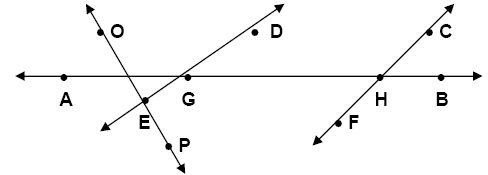Which point is on line DE?
 a. P d. G b. A e. H c. C

2.a. Ray AB d.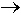AB b. Line AB e. Line segment AB c.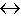AB

3.

Geometry – Shapes and Figures – RIT 181 – 190
Name these figures from left to right.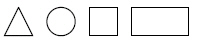a. circle, square, rectangle, triangle d. triangle, circle, square, rectangle b. square, rectangle, triangle, circle e. triangle, circle, rectangle, square c. rectangle, triangle, circle, square

4.

Which is a cube?
 a.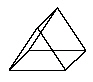d.b.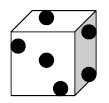e.c.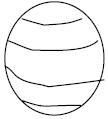5.

What flat shape has 3 sides and 3 corners?
 a. cube d. rectangle b. triangle e. sphere c. square

6.

Geometry – Symmetry and Transformations – RIT 181 – 190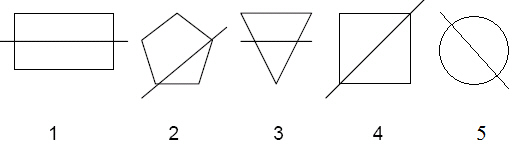Which figures show a line of symmetry?
 a. 1, 4, and 5 d. 1 and 4 b. 2, 4, and 5 e. 2, 3, 4 c. 4 and 5

7.Which pairs of objects have been turned?
 a. 1, 4, and 5 d. 2 and 3 b. 3 and 4 e. 3, 4, and 5 c. 1, 2, and 3

8.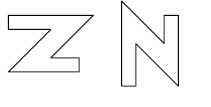The figure on the left has been moved to get the figure on the right. This is an
example of _____.
 a. symmetry d. congruency b. flip e. slide c. turn

9.

Geometry – Congruency and Similarity – RIT 181 – 190
Which angles are congruent?
 a.c.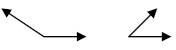b.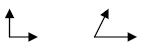d.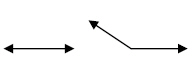10.

Which figures are similar, but not congruent?
 a.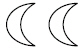d.b.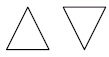e.c.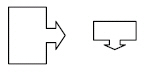11.

Geometry – Shapes and Figures – RIT 191 – 200
Find the sphere.
 a.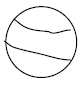d.b.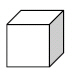e.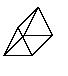c.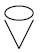12.a. cylinder d. sphere b. cone e. pyramid c. cube

13.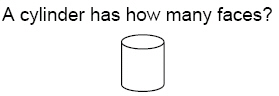a. 2 d. 4 b. 0 e. 1 c. 3

14.

Geometry – Symmetry and Transformations – RIT 191 – 200Which figure or figures show(s) a line of symmetry?
 a. 2, 3, and 4 d. 2 and 4 b. 2 only e. 3, 4, and 5 c. 1 and 2

15.

Geometry – Geometric Properties & Terminology – RIT 191 – 200
Which letter is in the middle?
A B C D E F G
 a. E d. G b. C e. D c. A